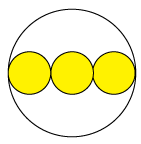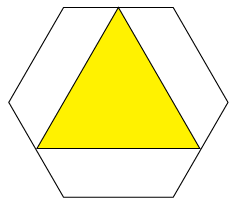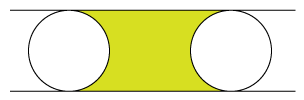# 10ticks Friday PuzzleWelcome to Ian’s Friday Puzzle! Dust off those Friday cobwebs with a little manipulation of the old grey matter. Perplexing puzzles, logical, illogical, and sometimes just plain stupid. Be prepared to be bewildered, befuddled and bedazzled!

See the latest Friday Puzzle below and look at all the past puzzles. Get the Friday Puzzle straight to your phone, follow us on Twitter. The first 5 correct answers join our Hall of Fame!

#### This week's Friday Puzzle

A circle has three equal smaller circles whose centres

are placed on its diameter as shown.Which area is bigger, the three shaded circles or

one of the two equal unshaded areas?

#### Friday Puzzle (26/11/2021)

Colin and his daughters Abigail and Brenda have the same birthday.

Today, Colin is 28, Abigail is 6 and Zoey is 2.

How old will Colin be when his age is the sum of the ages of Abigail and Brenda?

#### Friday Puzzle (19/11/2021)

The diagram shows an equilateral triangle inside a regular hexagon.The hexagon has sides of length 10 cm.

The vertices of the triangle are midpoints of sides of the hexagon.

What is the length of the perimeter of the triangle?

#### Friday Puzzle (12/11/2021)

Two circles of radius 2 cm fit exactly between two parallel lines, as shown in the diagram.The centres of the circles are 6 cm apart.

What is the area that is shaded yellow?

#### Friday Puzzle (05/11/2021)

A positive integer has exactly eight factors.

Two of these factors are 14 and 22.

What is the sum of all eight factors of the positive integer?

#### Friday Puzzle (29/10/2021)

The England T20 Cricket team are messing about.

Jason thinks of a positive integer, which Jos then doubles.

Dawid then trebles Jos’ number.

Finally Jonny multiplies Dawid’s number by six.

Eoin notices that the sum of the four numbers is a perfect square!

What is the smallest number that Jason could have thought of?

#### Friday Puzzle (22/10/2021)The diagram shows a regular hexagon with an area of 60 cm2.

What is the area of the shaded triangle?

#### Friday Puzzle (15/10/2021)

There are 243 players taking part in a knock-out tournament.

Each match in the tournament involves 3 players and only the winner of the match remains in the tournament − the other two players are knocked out.

How many matches are required until there is an overall winner?

#### Friday Puzzle (08/10/2021)

My biscuit barrel contains jammy dodgers and chocolate digestives.
The ratio of jammy dodgers to chocolate digestives in the barrel is 3 : 8.
When I remove one jammy dodger the ratio changes to 1 : 3.
How many chocolate digestives are in the basket?

#### Friday Puzzle (01/10/2021)

Solve (1 + 2 + 3 + 4 + 5 + 6 + 7 + 8 + 9 + 10)2 − (1 + 2 + 3 + 4 + 5 + 6 + 7 + 8 + 9)2

by starting with something smaller and looking for patterns.

(1 + 2)2 − (1 )2
(1 + 2 + 3)2 − (1 + 2)2
(1 + 2 + 3 + 4)2 − (1 + 2 + 3)2